# Inserting A Pivot Calculated Field In Microsoft Excel 2010

In this article, we will learn how to insert a excel calculated field.

We can insert calculated field in pivot table. This will allow us to calculate the result in a smoother way.

To calculate the bonus in our example, we will use IF function.

The IF function checks if the condition you specify is TRUE or FALSE. If the condition returns TRUE, then it returns preset value, and if the condition returns FALSE, then it returns another preset value.

Syntax = IF(logical_test,value_if_true,value_if_false)

logical_test: Logical test will test the condition or criteria. If the condition meets then it returns the preset value, and if the condition does not meet, then it returns another preset value.

value_if_true: The value that you want to be returned if this argument returns TRUE.

value_if_false: The value that you want to be returned if this argument returns FALSE

Let us take an example:

• We have employees in column A, production done in column B, salary in column C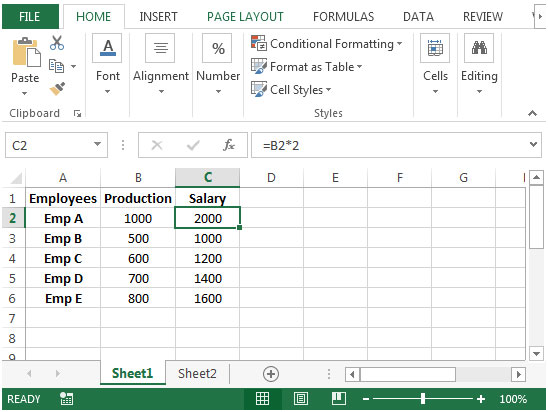• In column C, salary is calculated after multiplying the production done by employees with 2.
• From sheet1, we have created a pivot table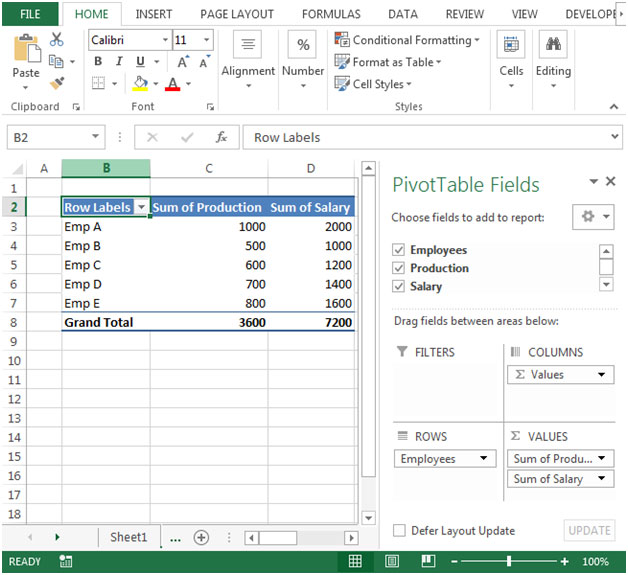• Click on any cell in Pivot table, and you will see Analyze ribbon will be activated.
• Click on Analyze ribbon
• In Calculations group, click on “Fields, Items, & Sets” option & click “Calculated Field”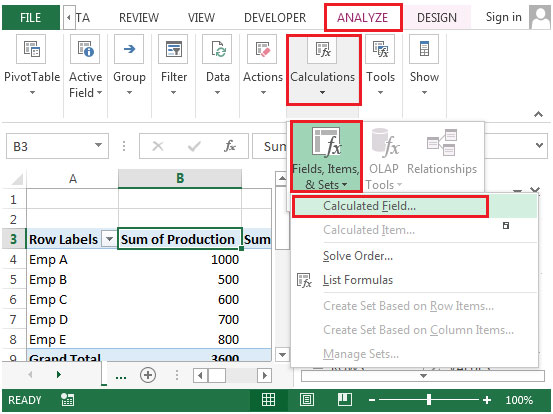• The following dialog box will appear.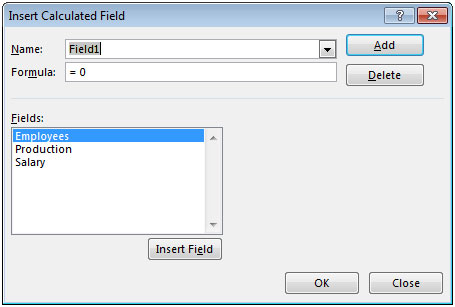• In Name box, enter the name for the field.
• In formula box, enter the formula as=IF( Production>600, Salary+( Salary*10%), Salary)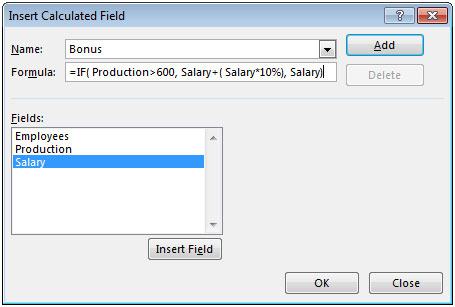• Click on Add button & then click on OK.
• You will see the new field as “Bonus” will appear in pivot table.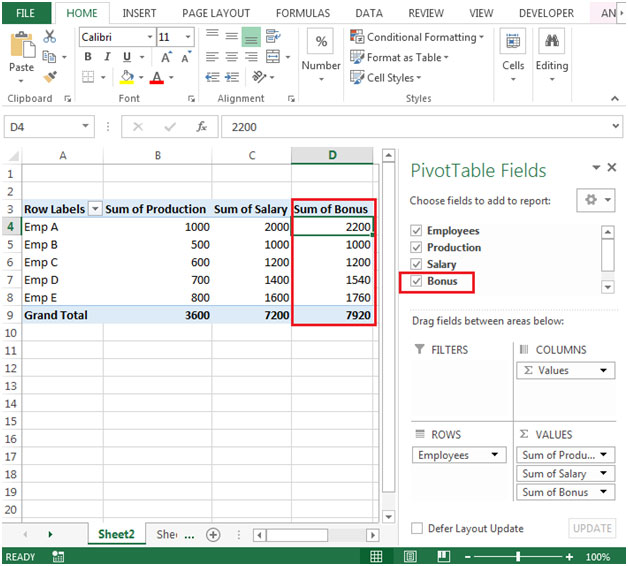In this way we can insert a calculated field in pivot table.

Terms and Conditions of use

The applications/code on this site are distributed as is and without warranties or liability. In no event shall the owner of the copyrights, or the authors of the applications/code be liable for any loss of profit, any problems or any damage resulting from the use or evaluation of the applications/code.